MORE IN Structural Analysis 1
VTU Civil Engineering (Semester 4)
Structural Analysis 1
June 2014
Total marks: --
Total time: --
INSTRUCTIONS
(1) Assume appropriate data and state your reasons
(2) Marks are given to the right of every question
(3) Draw neat diagrams wherever necessary

Differentiate between:
1 (a) (i) Determinate and indeterminate structures.
3 M
1 (a) (ii) Degree of redundancy and degree of freedom.
3 M
1 (a) (iii) Linear structure and non-linear structure.
3 M
1 (a) (iv) Deflection and deformation.
3 M
1 (b) Determine the strain energy stored in a member when it is subjected to an axial load and strain energy stored in beam subjected to bending moment. Use standard notations.
10 M

2 (a) Find the maximum slope and deflection for the beam shown in Fig. Q2(a) using moment area method. Take EI=10.2×103 kNm2.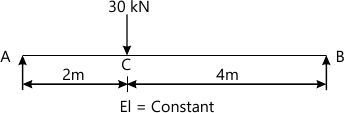10 M
2 (b) Find the slope and deflection at free end of a cantilever beam shown in Fig. Q2(b) using conjugate beam method. Take EI=2.5×106 kNm2.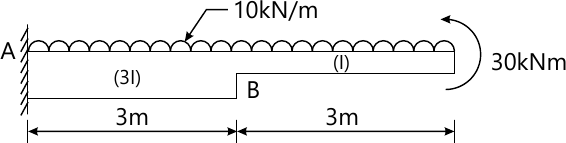10 M

3 (a) Determine the deflection for a simply supported beam shown in Fig. Q3 (a) at midspan using strain energy method. Take EI=3×104 kNm2.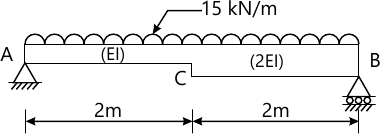10 M
3 (b) Determine the deflection for a simply supported beam shown in Fig. Q3 (b) under the point load by strain energy method. Take E=200×106 kN/m2 and I=25×10-6 m4.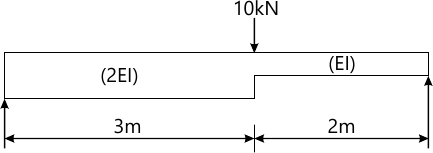10 M

4 (a) Analyse the fixed beam shown in Fig.Q4(a) by strain energy method. Also draw B.M.D.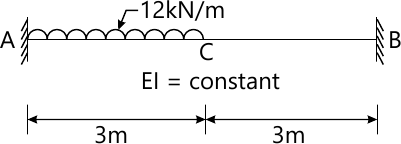10 M
4 (b) Analyse the fixed beam shown in Fig.Qa(b) by strain energy method. Also draw B.M.D.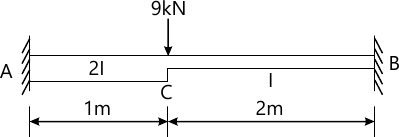10 M

5 (a) A three hinged symmetrical arch of span 20 m, hinges are provided at supports and crown of the arch. The rise at crown is 5 m and arch is subjected to a point load of 200 kN at 6 m from the left support. Find the reactions at supports and calculate normal thrust and radial shear at 6 m from left support. Draw the bending moment diagram and also indicate the position of maximum positive and negative bending moment.
10 M
5 (b) A cable is supported on piers at 80 m apart at the same level has a central dip of 8m. Calculate maximum tension in the cable, when it is subjected to UDL, of 50 kN/m throughout the length. Also determine the vertical force on pier. If the back stay is inclined at 60° to the vertical when (i) the cable passes over a pulley, (ii) the cable passes over a saddle.
10 M

6 (a) Analyse the propped cantilever beam shown in Fig. Q6(a), using consistent deformation method, if support B sinks by 10 mm. Take EI=40000 kNm2. Also draw the BMD and SFD.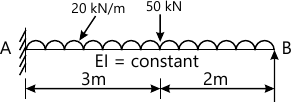10 M
6 (b) Analyse the fixed beam shown in Fig. Q6(b) using consistent deformation method. Draw the BMD and SFD.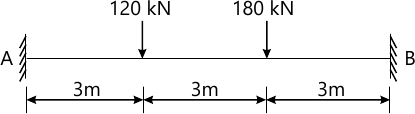10 M

7 Analyse the continuous beam shown in Fig. Q7 using three moment equation and draw bending moment diagram and shear force diagram. Also indicate the position of maximum positive and negative bending moment in the span AB and BC.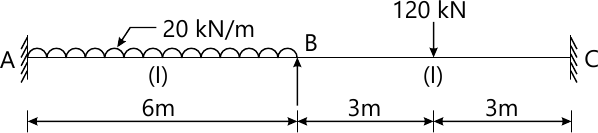20 M

8 A two hinged parabolic arch of span 24m and rise 3m is uniformly loaded over the left half of the span with 30 kN/m and a concentrated load of 70 kN at the crown. Determine the horizontal thrust assuming secant variation for moment of inertia.
20 M

More question papers from Structural Analysis 1